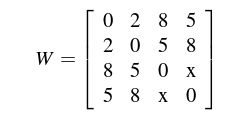# GATE | GATE-CS-2016 (Set 1) | Question 48

• Difficulty Level : Medium
• Last Updated : 19 Nov, 2018

Consider the weighted undirected graph with 4 vertices, where the weight of edge {i, j} g is given by the entry
Wij in the matrix WThe largest possible integer value of x, for which at least one shortest path between some pair of vertices will contain the edge with weight x is ________

Note : This question was asked as Numerical Answer Type.

(A) 8
(B) 12
(C) 10
(D) 11